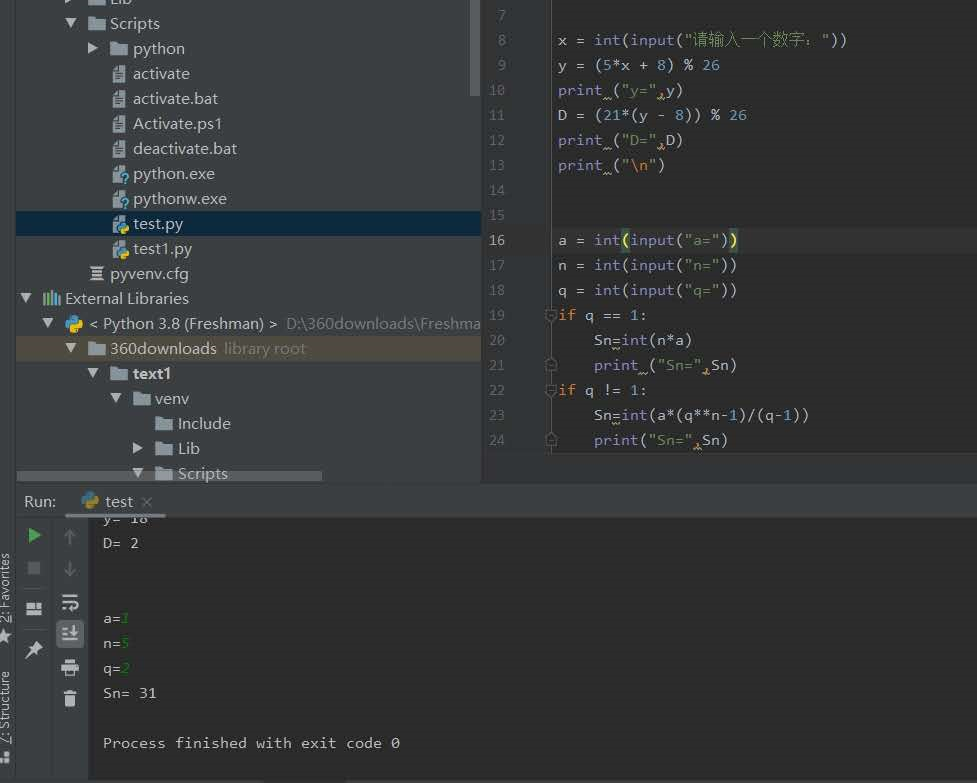# 第一次实验报告

## 1.实验内容

1．熟悉Python开发环境；
2．练习Python运行、调试技能；
3．编写程序，练习变量和类型、字符串、对象、缩进和注释等；
4．掌握git技能

## 2.实验过程及结果

x = int(input("请输入一个数字："))
y = (5*x + 8) % 26
print ("y=",y)
D = (21*(y - 8)) % 26
print ("D=",D)
print ("\n")

a = int(input("a="))
n = int(input("n="))
q = int(input("q="))
if q == 1:
Sn=int(n*a)
print ("Sn=",Sn)
if q != 1:
Sn=int(a*(q**n-1)/(q-1))
print("Sn=",Sn)## 3.实验过程中遇到的问题和解决过程

问题1：完成编写代码后不能正确运行。

问题1解决办法：debug程序，分步调试后发现if语句的语法用错了，没有加分号。

• 问题2：之前的提交作业中遇到对知识点（比如元组）掌握不牢固，无法完成程序编写。
• 问题2解决办法：通过百度去找寻办法。

4.其他（感悟、思考等）

python其实和其他语法还是有一些差距的，基本的程序语法是明白的，但是还是对于python里的if，while语句不太清晰，毕竟接触的比较少，更多的是c和c++，需要去百读多看看其他人的方法。

posted @ 2020-07-06 13:28  Mryuss  阅读(45)  评论(0编辑  收藏